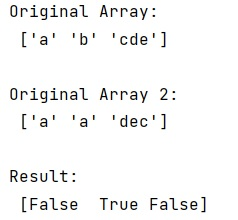# numpy.char.compare_chararrays() Method with Example

Learn about the numpy.char.compare_chararrays() method with its usage, syntax, and example.
Submitted by Pranit Sharma, on March 21, 2023

## numpy.char.compare_chararrays() Method

The numpy.char.compare_chararrays() is used to perform the element-wise comparison of two string arrays. There are different types of comparisons that can be performed based on the type of comparison operator passed as a parameter (cmp).

## Syntax

```char.compare_chararrays(a1, a2, cmp, rstrip)
```

## Parameter(s)

• a1, a2: input arrays
• cmp: type of comparison ("<", "<=", "==", ">=", ">", "!=").
• rstrip: A Boolean value, True or False, indicating whether spaces at the end of strings are removed before the comparison or not.

## Return Value

The char.compare_chararrays() method returns a Boolean type array with the same size as the two input arrays passed to it.

## Python code to demonstrate the example of numpy.char.compare_chararrays() method

```# Import numpy
import numpy as np

# Crating two numpy arrays
arr = np.array(["a", "b", "cde"])
arr1 = np.array(["a", "a", "dec"])

# Display original arrays
print("Original Array:\n",arr,"\n")
print("Original Array 2:\n",arr1,"\n")

# Comparing both arrays element-wise
res = np.compare_chararrays(arr,arr1,">", True)

# Display result
print("Result:\n",res,"\n")
```

### Output